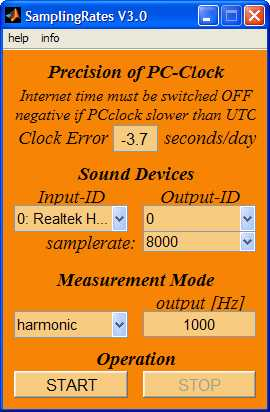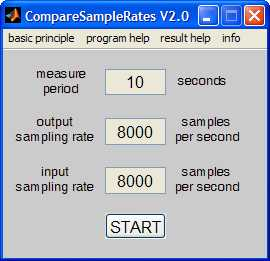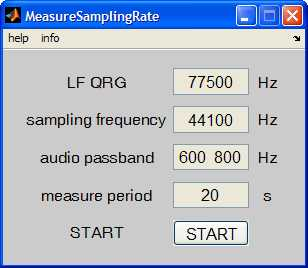# Tools to Measure Samplingrates

It is unbelievable, but the samplerates of PCs are the most inaccurate part of a modern hamradio station. You can lock your rig to GPS. But frequencies can differ by several Hertz simply because the the samplerate is less accurate than a sandglass.

The following programs differ in respect to the reference clock (internal PC-clock, external counter, longwave frequency normal)

## samplingrates

This program measures the deviation between the PC-system clock and the clocks of the sampling process. The sampling clock is defined by ts = #samples/fs, where #samples is the number of samples acquired, and fs is the nominal sampling rate (for example 44100). To get absolute samplerates, the error of the system clock (in seconds per day) must be known.

When the program is started the clocks are synchronized. At increasing time the clocks will diverge. To get an accurate measure of the actual sampling rates, the time between Start and Stop of the program should be of the order of 15 minutes. Very much larger times are possible to detect variabilities in the frequencies.## comparesamplerates

This program measures the deviation between the sampling rates of sound output and sound input.

The basic method is simple:

(1) A sine wave of 1600 Hz is generated on the output. The analog output of the sound card is fed back into the analog input of the sound card. This feed back must be installed by an appropriate audio cable.

(2) The program measures the phase difference between outgoing and incoming sine wave as a function of time.

(3) The change of the phase difference is transformed to a corresponding error of the sample rate and to an accumulating clock error.## measuresamplingrate

This program only works in cooperation with a Longwave receiver and an easy-to-build small hardware. The principle is simple:

A frequency normal is received on longwave, for example DCF77 on 77500 Hz. Soundcards running at a sampling frequency fs can only generate sine waves of frequencies f lower than half of the sampling frequency.

If such an analog sine wave is distorted by a non-linear element such as a diode then harmonics 2*f, 3*f, 4*f, 5*f,... are produced and can be heard by the long wave receiver. Often the odd harmonics are stronger. If both, the odd harmonic and the frequency normal are received in SSB or CW mode within the selected bandwidth of the receiver then the resulting audio signal can be analyzed by the computer (running this program).

To measure fs simply f must be chosen such that one of its odd harmonics matches fs. To measure fs = 8000 Hz using the frequency normal 77500 Hz f must be considerably lower than 4000 Hz and it must divide 77500. This program automatically takes the largest f = fs/n for odd n that is smaller than 0.4*fs. In the case of fs=8000 Hz this results in f = 3200 Hz (n=25).